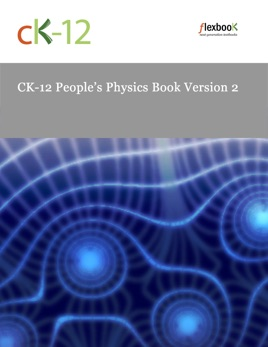## Publisher Description

CK-12 Foundation's People’s Physics Book Version 2 Covers the following chapters: Units and Problem Solving- Units, conversions, measurements & symbols Energy Conservation- 5 laws One-Dimensional Motion- motion, velocity, acceleration & deceleration Two Dimensional and Projectile Motion- motion & velocity Newton’s Laws- study of motion, acceleration & force Centripetal Forces- study of force, velocity changes, net-forces, applied forces & acceleration Momentum Conservation- study of motion, mass, velocity, force of gravity & collision Energy and Force- laws of conservation of energy & force Rotational Motion- Conservation of angular momentum, rotational velocity, rotational inertia/motion, , torque & linear motion Simple Harmonic Motion- SMH, periodic motion, oscillation, restorative force, frequency, amplitude & kinetic energy Wave Motion and Sound- harmonic motion, longitudinal/transverse & sound/water waves & interference Electricity- conservation of charge, electromagnetism, charge, gravity, potential energy & electric force Electric Circuits: Batteries and Resistors- Ohm's Law, electric currents/fields, voltage, current, resistance & AC/DC power Magnetism- electromagnetic force, Coulomb electric force, electric current, magnetic fields, electricity & right/left hand rules Electric Circuits: Advanced Topics- circuitry, diodes, inductors, transistors, amplifiers & chips Lights- waves, electromagnetic radiation/ fields, electrons, photons, Fermat’s Principle, refraction/diffraction, scattering/color absorption & dispersion Fluids- force, momentum, density, Archimedes’, Pascal’s & Bernoulli’s Principles Thermodynamics and Heat Engines- Adiabatic process, thermodynamics & entropy Gas Laws Heat Engines and the Laws of Thermodynamics BCTherm Special and General Relativity- Theory of Relativity, Lorentz time dilation/length contraction, speed of light, space time, black holes & event horizons Radioactivity and Nuclear Physics- fission/fusion & radioactive decay Standard Model of Particle Physics- subatomic particles, Standard Model, CPT symmetry, fermions, bosons, leptons, quarks & fundamental forces
Feynman's Diagrams- drawing Feynman’s Diagrams Quantum Mechanics- wave-particle duality The Physics of Global Warming– radiation & thermodynamics Answers to Selected Problems Equations and Fundamental Constants

## Customer Reviews

Kkannik98 ,

### Good Information

This book did benefit me along the lines of basic physics, but it lacks some important topics. I also found many grammatical spelling errors. For that I felt more uneasy about trusting this book.